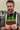Margin and Padding SCSS Mixins for Grid Alignment

Horizontal and vertical flow are important in making an app's appearance easy to read and pleasant to look at. I wanted to create an SCSS mixin that helps me unify all padding and margin to a base unit of size for a consistent layout and also mimic the css shorthand.

Final Results

\$base-font-size: 14;
\$x-height: 8;
\$baseline: 4;

\$spacing-size: \$baseline;

/**
Calculate spacing in rems based off multiples of fine grid size
**/
@function calcSpacingRems(\$multiple) {
@return #{\$multiple * (\$spacing-size / \$base-font-size)}rem;
}

/**
Add margin(s) to element based on multiples of \$fine-grid-size in rems. Uses the shorthand
syntax. Ex: margin(1), margin(1,2), margin(1,2,3), margin(1,2,3,4)
**/
@mixin margin(\$multipliers...) {
\$content: null;
@for \$i from 1 through length(\$multipliers) {
\$val: nth(\$multipliers, \$i);
@if \$val == auto {
\$content: append(\$content, auto);
} @else {
\$content: append(\$content, calcSpacingRems(nth(\$multipliers, \$i)));
}
}
margin: \$content;
}

/**
Add padding(s) to element based on multiples of \$fine-grid-size in rems. Uses the shorthand
**/
\$content: null;
@for \$i from 1 through length(\$multipliers) {
\$content: append(\$content, calcSpacingRems(nth(\$multipliers, \$i)));
}
}

/**
A more generalized way to add spacing, pass in property name and multiplier
**/
#{\$property}: calcSpacingRems(\$multiplier);
}

Explanation

Calculating Spacing

The app currently uses a base font size of 14px with a font x-height of 8px and a baseline of 4px. The unit spacing size is based on the baseline value, so everything will be spaced in multiples of 4. Let’s first define some variables and create a function that calculates the padding based on the multiplier and converts the value to rems.

\$base-font-size: 14;
\$x-height: 8;
\$baseline: 4;

\$spacing-size: \$baseline;

@function calcSpacingRems(\$multiple) {
@return #{\$multiple * (\$spacing-size / \$base-font-size)}rem;
}

We divide the spacing size by the base font size to get the base value in rems, then multiply the value by he multiplier.

Now that we can calculate the spacing, let’s create a mixin that will return a margin.

Goals

• I want to mimic the shorthand functionality of margin that can accept 1, 2, 3 or 4 values. (w3schools.com/css/css_margin.asp)
• I need to handle auto if it’s passed in
• The arguments are the multiplier values
• Returns a value in rems
@mixin margin(\$multipliers...) {
\$content: null;
@for \$i from 1 through length(\$multipliers) {
\$val: nth(\$multipliers, \$i);
@if \$val == auto {
\$content: append(\$content, auto);
} @else {
\$content: append(\$content, calcSpacingRems(nth(\$multipliers, \$i)));
}
}
margin: \$content;
}

The ... syntax in the argument definition allows any number of arguments to be added to the function. Since there could be 1 to 4 arguments, we can loop through them using the scss @for syntax and process each one. On each loop, if the value is auto we skip the processing and use auto, otherwise we use the calcSpacingRems() function to convert the multiplier integer to a rem value. We then use the SCSS append() function to concatenate valid css values to the \$content variable. Once it’s finished looping through the list, we just return the calculated margin css property.

We can repeat the same process for the padding, but since auto is not valid syntax, we can skip the conditional.

\$content: null;
@for \$i from 1 through length(\$multipliers) {
\$content: append(\$content, calcSpacingRems(nth(\$multipliers, \$i)));
}
}

Adding Spacing in a Single Direction

The padding and margin mixins require 4 arguments to add a value in a single direction (Ex: padding(1, 0, 0, 0)). To make it easier, I created a simple mixin that takes the spacing direction property and a single value as arguments.

#{\$property}: calcSpacingRems(\$multiplier);
}

For example, to add a top margin, use addSpacing('margin-top', 2) instead of padding(2, 0, 0, 0). I’m not sure if it’s cleaner, but it is easier to know what property is being changed at a glance.

Riding the Happy Path

As you may have noticed, I don’t have any error messaging or catches for incorrect arguments values, I’m just assuming everyone knows what they’re doing. SCSS does provide great ways to handle this, but I just kept the complexity simple for this demonstration. Feel free to add on and improve!